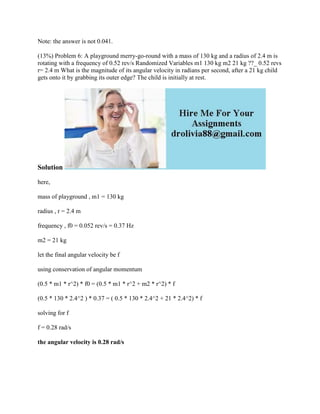Diese Präsentation wurde erfolgreich gemeldet.

# Note- the answer is not 0-041- (13-) Problem 6- A playground merry-go-.docx

Anzeige
Anzeige
Anzeige
Anzeige
Anzeige
Anzeige
Anzeige
Anzeige
Anzeige
Anzeige
Anzeige×

1 von 2 Anzeige

# Note- the answer is not 0-041- (13-) Problem 6- A playground merry-go-.docx

Note: the answer is not 0.041.
(13%) Problem 6: A playground merry-go-round with a mass of 130 kg and a radius of 2.4 m is rotating with a frequency of 0.52 rev/s Randomized Variables m1 130 kg m2 21 kg ??_ 0.52 revs r= 2.4 m What is the magnitude of its angular velocity in radians per second, after a 21 kg child gets onto it by grabbing its outer edge? The child is initially at rest.
Solution
here,
mass of playground , m1 = 130 kg
radius , r = 2.4 m
frequency , f0 = 0.052 rev/s = 0.37 Hz
m2 = 21 kg
let the final angular velocity be f
using conservation of angular momentum
(0.5 * m1 * r^2) * f0 = (0.5 * m1 * r^2 + m2 * r^2) * f
(0.5 * 130 * 2.4^2 ) * 0.37 = ( 0.5 * 130 * 2.4^2 + 21 * 2.4^2) * f
solving for f
the angular velocity is 0.28 rad/s
.

Note: the answer is not 0.041.
(13%) Problem 6: A playground merry-go-round with a mass of 130 kg and a radius of 2.4 m is rotating with a frequency of 0.52 rev/s Randomized Variables m1 130 kg m2 21 kg ??_ 0.52 revs r= 2.4 m What is the magnitude of its angular velocity in radians per second, after a 21 kg child gets onto it by grabbing its outer edge? The child is initially at rest.
Solution
here,
mass of playground , m1 = 130 kg
radius , r = 2.4 m
frequency , f0 = 0.052 rev/s = 0.37 Hz
m2 = 21 kg
let the final angular velocity be f
using conservation of angular momentum
(0.5 * m1 * r^2) * f0 = (0.5 * m1 * r^2 + m2 * r^2) * f
(0.5 * 130 * 2.4^2 ) * 0.37 = ( 0.5 * 130 * 2.4^2 + 21 * 2.4^2) * f
solving for f